# Are different notions of the crossing number the same?

 Importance: High ✭✭✭
 Author(s): Pach, János Tóth, Géza
 Subject: Graph Theory » Topological Graph Theory » » Crossing numbers
 Keywords: crossing number pair-crossing number
 Posted by: cibulka on: November 3rd, 2009
Problem   Does the following equality hold for every graph?The crossing number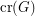of a graphis the minimum number of edge crossings in any drawing ofin the plane. In the pairwise crossing number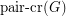, we minimize the number of pairs of edges that cross.

Obviously we have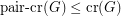.

The problem was first posed by Pach and Tóth in~[PT], who first spotted the possibility that the pairwise crossing number might be different from the crossing number. They proved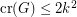for graphs with pairwise crossing number, which was later improved by Valtr~[V05] to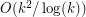and by Tóth~[T08] to.

## Bibliography

*[PT] János Pach, Géza Tóth, Which crossing number is it anyway?, Journal of Combinatorial Theory Series B 80 (2000), no. 2, 225--246. MathSciNet

[V05] Pavel Valtr, On the pair-crossing number, Combinatorial and computational geometry, 52 (2005), 569--575. MathSciNet

[T08] Géza Tóth, Note on the pair-crossing number and the odd-crossing number, Discrete Comput. Geom., 39 (2008), no. 4, 791--799. MathSciNet

* indicates original appearance(s) of problem.